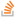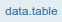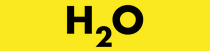# Home

`data.table` is one of the 15,000 add-on packages for the programming language R which is popular in these fields. It provides a high-performance version of base R's `data.frame` with syntax and feature enhancements for ease of use, convenience and programming speed. As of Nov 2018, data.table was the 4th largest Stack Overflow tag about an R package with over 8,000 questions, the 10th most starred R package on GitHub and had over 650 CRAN and Bioconductor packages using it. Here are a further 6,000 accepted answers which use/mention data.table but where the question was not specifically about data.table.

We have updated the 2014 grouping benchmarks comparing data.table to pandas and dplyr, and included Spark and pydatatable. The benchmark is automated and runs regularly against the latest versions of these packages. It is a work in progress: h2oai.github.io/db-benchmark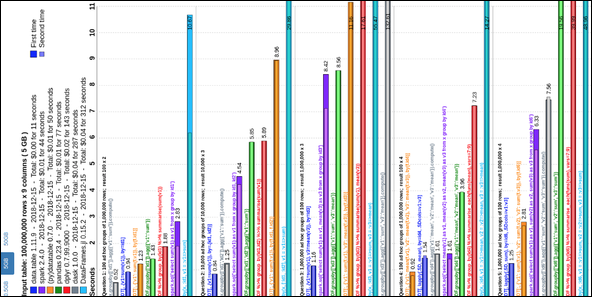These queries can be chained together just by adding another one on the end:
`DT[...][...]`
See data.table compared to dplyr on Stack Overflow and Quora.

``````> require(data.table)
> example(data.table)

# basic row subset
DT                                       # 2nd row
DT[2:3]                                     # 2nd and 3rd row
w=2:3; DT[w]                                # same
DT[order(x)]                                # no need for DT\$ prefix on column x
DT[order(x), ]                              # same; the ',' is optional
DT[y>2]                                     # all rows where DT\$y > 2
DT[y>2 & v>5]                               # compound logical expressions
DT[!2:4]                                    # all rows other than 2:4
DT[-(2:4)]                                  # same

# select|compute columns
DT[, v]                                     # v column (as vector)
DT[, list(v)]                               # v column (as data.table)
DT[, .(v)]                                  # same; .() is an alias for list()
DT[, sum(v)]                                # sum of column v, returned as vector
DT[, .(sum(v))]                             # same but return data.table
DT[, .(sv=sum(v))]                          # same but name column "sv"
DT[, .(v, v*2)]                             # return two column data.table

# subset rows and select|compute
DT[2:3, sum(v)]                             # sum(v) over rows 2 and 3
DT[2:3, .(sum(v))]                          # same, but return data.table
DT[2:3, .(sv=sum(v))]                       # same, but name column "sv"
DT[2:5, cat(v, "\n")]                       # just for j's side effect

# select columns the data.frame way
DT[, 2]                                     # 2nd column, a data.table always
colNum = 2
DT[, ..colNum]                              # same as DT[,2]; ..var => one-up
DT[["v"]]                                   # same as DT[,v] but lower overhead

# grouping operations - j and by
DT[, sum(v), by=x]                          # appearance order of groups preserved
DT[, sum(v), keyby=x]                       # order the result by group
DT[, sum(v), by=x][order(x)]                # same by chaining expressions together

# fast ad hoc row subsets (subsets as joins)
DT["a", on="x"]                             # same as x == "a" but uses key (fast)
DT["a", on=.(x)]                            # same
DT[.("a"), on="x"]                          # same
DT[x=="a"]                                  # same, == internally optimized
DT[x!="b" | y!=3]                           # not yet optimized
DT[.("b", 3), on=c("x", "y")]               # same as DT[x=="b" & y==3]
DT[.("b", 3), on=.(x, y)]                   # same
DT[.("b", 1:2), on=c("x", "y")]             # no match returns NA
DT[.("b", 1:2), on=.(x, y), nomatch=0]      # no match row is not returned
DT[.("b", 1:2), on=c("x", "y"), roll=Inf]   # locf, previous row rolls forward
DT[.("b", 1:2), on=.(x, y), roll=-Inf]      # nocb, next row rolls backward
DT["b", sum(v*y), on="x"]                   # same as DT[x=="b", sum(v*y)]

# all together
DT[x!="a", sum(v), by=x]                    # get sum(v) by "x" for each i != "a"
DT[!"a", sum(v), by=.EACHI, on="x"]         # same, but using subsets-as-joins
DT[c("b","c"), sum(v), by=.EACHI, on="x"]   # same
DT[c("b","c"), sum(v), by=.EACHI, on=.(x)]  # same, using on=.()

# joins as subsets
X = data.table(x=c("c","b"), v=8:7, foo=c(4,2))
X

DT[X, on="x"]                               # right join
X[DT, on="x"]                               # left join
DT[X, on="x", nomatch=0]                    # inner join
DT[!X, on="x"]                              # not join
DT[X, on=c(y="v")]                          # join DT\$y to X\$v
DT[X, on="y==v"]                            # same

DT[X, on=.(y<=foo)]                         # non-equi join
DT[X, on="y<=foo"]                          # same
DT[X, on=c("y<=foo")]                       # same
DT[X, on=.(y>=foo)]                         # non-equi join
DT[X, on=.(x, y<=foo)]                      # non-equi join
DT[X, .(x,y,x.y,v), on=.(x, y>=foo)]        # select x's join columns as well

DT[X, on="x", mult="first"]                 # first row of each group
DT[X, on="x", mult="last"]                  # last row of each group
DT[X, sum(v), by=.EACHI, on="x"]            # join and eval j for each row in i
DT[X, sum(v)*foo, by=.EACHI, on="x"]        # join inherited scope
DT[X, sum(v)*i.v, by=.EACHI, on="x"]        # 'i,v' refers to X's v column
DT[X, on=.(x, v>=v), sum(y)*foo, by=.EACHI] # non-equi join with by=.EACHI

# setting keys
kDT = copy(DT)                              # copy DT to kDT to work with it
setkey(kDT,x)                               # set a 1-column key.
setkeyv(kDT,"x")                            # same (v in setkeyv stands for vector)
v="x"
setkeyv(kDT,v)                              # same
key(kDT)                                    # "x"

# fast *keyed* subsets
kDT["a"]                                    # subset-as-join on *key* column 'x'
kDT["a", on="x"]                            # same, being explicit using 'on='

# all together
kDT[!"a", sum(v), by=.EACHI]                # get sum(v) for each i != "a"

# multi-column key
setkey(kDT,x,y)                             # 2-column key
setkeyv(kDT,c("x","y"))                     # same

# fast *keyed* subsets on multi-column key
kDT["a"]                                    # join to 1st column of key
kDT["a", on="x"]                            # on= is optional but preferred
kDT[.("a")]                                 # same; .() is an alias for list()
kDT[list("a")]                              # same
kDT[.("a", 3)]                              # join to 2 columns
kDT[.("a", 3:6)]                            # join 4 rows (2 missing)
kDT[.("a", 3:6), nomatch=0]                 # remove missing
kDT[.("a", 3:6), roll=TRUE]                 # locf rolling join
kDT[.("a", 3:6), roll=Inf]                  # same
kDT[.("a", 3:6), roll=-Inf]                 # nocb rolling join
kDT[!.("a")]                                # not join
kDT[!"a"]                                   # same

DT[.N]                                      # last row
DT[, .N]                                    # total number of rows in DT
DT[, .N, by=x]                              # number of rows in each group
DT[, .SD, .SDcols=x:y]                      # select columns 'x' and 'y'
DT[, .SD]                                # first row; same as DT[1,]
DT[, .SD, by=x]                          # first row of each group
DT[, c(.N, lapply(.SD, sum)), by=x]         # group size alongside sum
DT[, .I, by=x]                           # row number of first row of each group
DT[, grp := .GRP, by=x]                     # add a group counter column
X[, DT[.BY, y, on="x"], by=x]               # join within group to use less ram

# add/update/delete by reference (see ?assign)
print(DT[, z:=42L])                         # add new column by reference
print(DT[, z:=NULL])                        # remove column by reference
print(DT["a", v:=42L, on="x"])              # subassign to column
print(DT["b", v2:=84L, on="x"])             # subassign to new column (NA padded)

DT[, m:=mean(v), by=x][]                    # add new column by reference by group
# postfix [] is shortcut to print()
DT[, sum(v), by=.(y%%2)]                    # expressions in by
DT[, sum(v), by=.(bool = y%%2)]             # same with name for group expression
DT[, .SD, by=x]                          # get 2nd row of each group
DT[, tail(.SD,2), by=x]                     # last 2 rows of each group
DT[, lapply(.SD, sum), by=x]                # sum of all columns for each group
DT[, .SD[which.min(v)], by=x]               # nested query by group

DT[, list(MySum=sum(v),
MyMin=min(v),
MyMax=max(v)),
by=.(x, y%%2)]                          # by 2 expressions

DT[, .(a = .(a), b = .(b)), by=x]           # list columns
DT[, .(seq = min(a):max(b)), by=x]          # j is not limited to just aggregations
DT[, sum(v), by=x][V1<20]                   # compound query
DT[, sum(v), by=x][order(-V1)]              # ordering results
DT[, c(.N, lapply(.SD,sum)), by=x]          # group size and sums by group
DT[, {tmp <- mean(y);                       # anonymous lambda in 'j'; j any valid
.(a = a-tmp, b = b-tmp)               #   expression where every element
}, by=x]                              #   becomes a column in result

pdf("new.pdf")
DT[, plot(a,b), by=x]                       # can also plot in 'j'
dev.off()

# get max(y) and min of a set of columns for each consecutive run of 'v'
DT[, c(.(y=max(y)), lapply(.SD, min)), by=rleid(v), .SDcols=v:b]
``````Other features include :

• fast and friendly delimited file reader: `?fread`. It accepts system commands directly (such as `grep` and `gunzip`), has other convenience features for small data and is now parallelized on CRAN May 2018 and presented earlier here.
• fast and parallelized file writer: `?fwrite` announced here and on CRAN in Nov 2016.
• parallelized row subsets - See this benchmark for timings
• fast aggregation of large data; e.g. 100GB in RAM (see benchmarks on up to two billion rows)
• fast add/update/delete columns by reference by group using no copies at all
• fast ordered joins; e.g. rolling forwards, backwards, nearest and limited staleness
• fast overlapping range joins; similar to `findOverlaps` function from IRanges/GenomicRanges Bioconductor packages, but not limited to genomic (integer) intervals.
• fast non-equi (or conditional) joins, i.e., joins using operators `>, >=, <, <=` as well, available from v1.9.8+
• a fast primary ordered index; e.g. `setkey(DT,col1,col2)`
• automatic secondary indexing; e.g. `DT[col==val,]` and `DT[col %in% vals,]`
• fast and memory efficient combined join and group by; `by=.EACHI`
• fast reshape2 methods (dcast and melt) without needing reshape2 and its dependency chain installed or loaded
• group summary results may be many rows (e.g. first and last row by group) and each cell value may itself be a vector/object/function (e.g. unique ids by group as a list column of varying length vectors - this is pretty printed with commas)
• special symbols built-in for convenience and raw speed by avoiding the overhead of function calls: `.N`, `.SD`, `.I`, `.GRP` and `.BY`
• any R function from any R package can be used in queries not just the subset of functions made available by a database backend
• has no dependencies at all other than base R itself, for simpler production/maintenance
• the R dependency is as old as possible for as long as possible and we test against that version; e.g., v1.9.8 released on 25-Nov-2016 bumped the dependency up from 4.5 year old R 2.14.0 to 3 year old R 3.0.0.

Version 1.0 was released to CRAN in 2006. In June 2014 we moved from R-Forge to GitHub.Guidelines for filing issues / pull requests: Contribution Guidelines.

Wiki Home
Getting started
Events: Videos & Slides
Articles
Installation
Support
Contributing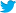#rdatatable@MattDowle@arun_sriniv